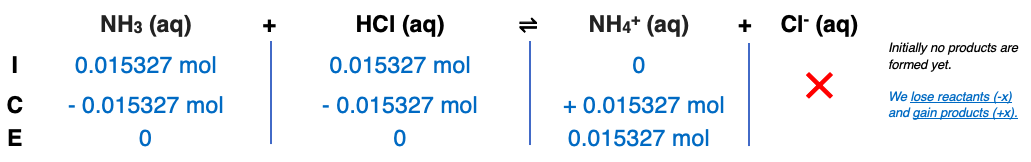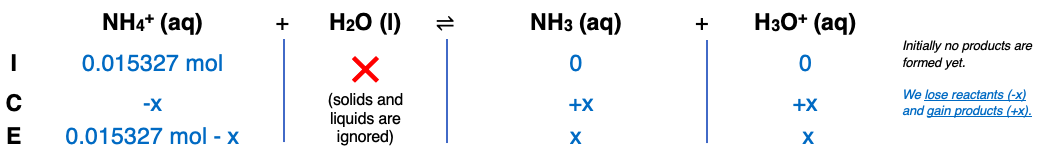# Problem: Find the pH of the equivalence point(s) and the volume (mL) of 0.125 M HCl needed to reach the point(s) in titrations of(a) 65.5 mL of 0.234 M NH 3

###### FREE Expert Solution

Step 1

At equivalence: mol acid = mol base

mol NH3 = 0.015327 mol NH3

volume HCl = 123 mL

Step 2Step 3Step 4

→ remove (-x)

x = 2.9510x10-6 mol

100% (351 ratings)###### Problem Details

Find the pH of the equivalence point(s) and the volume (mL) of 0.125 M HCl needed to reach the point(s) in titrations of

(a) 65.5 mL of 0.234 M NH 3